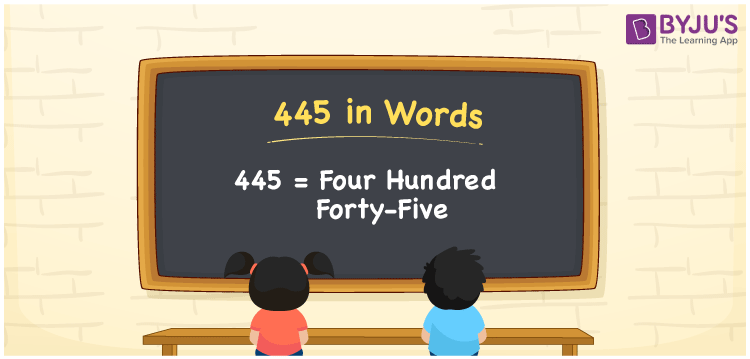# 445 in words

445 in words is written as Four Hundred and Forty Five. 445 represents the count or value. The article on Counting Numbers can give you an idea about count or counting. The number 445 is a 3 digit number that is used in expressions related to money, days, distance, length, weight and so on. Let us consider an example for 445. “The living room area is Four Hundred and Forty Five square meters”. Another example is “I have a collection of Four Hundred and Forty Five mickey mouse items.”

 445 in words Four Hundred and Forty Five Four Hundred and Forty Five in Numbers 445

## 445 in English Words## How to Write 445 in Words?

We can convert 445 to words using a place value chart. The number 445 has 3 digits, so let’s make a chart that shows the place value up to 3 digits.

 Hundreds Tens Ones 4 4 5

Thus, we can write the expanded form as:

4 × Hundred + 4 × Ten + 5 × One

= 4 × 100 + 4 × 10 + 5 × 1

= 445

= Four Hundred and Forty Five.

445 is the natural number that is succeeded by 444 and preceded by 446.

445 in words – Four Hundred and Forty Five.

Is 445 an odd number? – Yes.

Is 445 an even number? – No.

Is 445 a perfect square number? – No.

Is 445 a perfect cube number? – No.

Is 445 a prime number? – No.

Is 445 a composite number? – Yes.

## Solved Example

1. Write the number 445 in expanded form

Solution: 4 × 100 + 4 × 10 + 5 × 1

We can write 445 = 400 + 40 + 5

= 4 × 100 + 4 × 10 + 5 × 1.

## Frequently Asked Questions on 445 in words

Q1

### How to write the number 445 in words?

445 in words is written as Four Hundred and Forty Five.
Q2

### State True or False. 445 is divisible by 3?

False. 445 is not divisible by 3.
Q3

### Is 445 a perfect square number?

No. 445 is not a perfect square number.